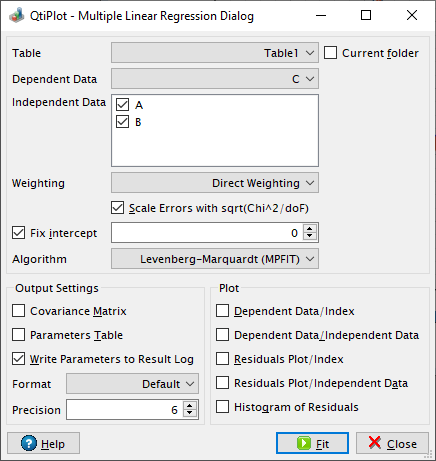# Multiple Linear Regression Dialog

This dialog box is opened when you select the Multiple Linear Regression... command from the Analysis -> Fitting menu. It allows to choose the dependent and independent data and the weighting method and to customize the output of the analysis. For more details about the algorithm used in QtiPlot please visit the Multiple Linear Regression Model section from the chapter dedicated to the Analysis of data and curves.

Figure 5-111. The Multiple Linear Regression dialog box.The available weighting methods are:

1. No weighting: all weighting coefficients are set to 1 (wi = 1).

2. Instrumental: the values of the associated error bars are used as weighting coefficients wi = 1/eri2, where eri are the error bar sizes stored in error bar columns. You must define Y error bar columns to the analyzed table before performing the fit.

3. Direct Weighting: the weighting coefficients are calculated as wi = yi, where yi are the y values in the dependent data set.

If the source data table contains Y error bar columns the weighting method is automatically set to Instrumental and a list box displays the names of the available Y error bar columns.

The Scale Errors with sqrt(Chi^2/doF) option becomes available only if the weighting method is set to something else than No weighting. This option only affects the errors on the parameters reported after the fit operation. It does not affect the fitting process or the data in any way. If checked, the reported errors on the parameters are calculated as the square root of the diagonal elements of the covariance matrix multiplied with sqrt(Chi2/(n - p)), where n is the number of data points and p the number of fit parameters. Otherwise, the reported errors equal the square root of the diagonal elements of the covariance matrix.

The numerical results of the fit operation will be displayed in the Log Panel if you check the output option Write Parameters to Result Log and/or in a new table if the option Parameters Table is checked. It is also possible to generate a Covariance Matrix window after each linear regression operation performed.

The options in the Plot group box can be used to specify the plot curves to be generated after each fit operation. If the Dependent Data/Index option is checked QtiPlot generates a scatter plot of the dependent data versus the data index (order of the data points). If the Dependent Data/Independent Data option is checked QtiPlot also generates a panel plot of the dependent data and of the predicted regression results with respect to each independent variable.

By default QtiPlot can generate three types of plots allowing to visualize the fit residuals. The residuals are calculated as the difference between the experimental Yi values and the response values predicted by the Multiple Linear Regression Model.

If the Residuals Plot/Index option is checked QtiPlot generates a scatter plot of the fit residuals versus the data index (order of the data points). If the Residuals Plot/Independent Data option is checked QtiPlot also generates a panel graphs window displaying the scatter plot of the fit residuals with respect to each independent variable. If the Histogram of Residuals option is checked QtiPlot creates a plot window displaying the histogram of the residuals.